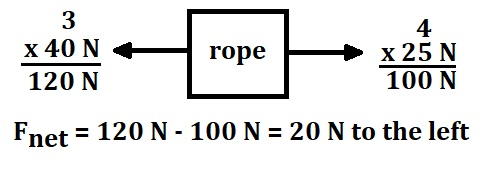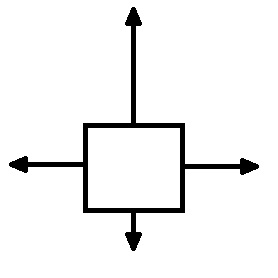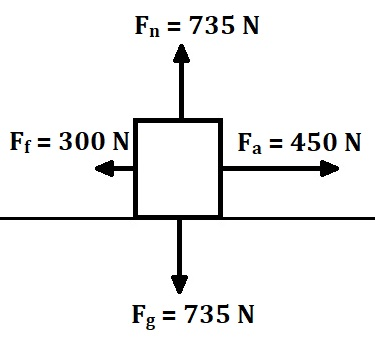# Newton's First, Second & Third Laws Flashcards

Newton's First, Second & Third Laws Flashcards
1/16 (missed) 0 0
Create Your Account To Continue Studying

As a member, you'll also get unlimited access to over 79,000 lessons in math, English, science, history, and more. Plus, get practice tests, quizzes, and personalized coaching to help you succeed.

Try it risk-free for 30 days. Cancel anytime
Proportionality and Newton's Second Law of Motion

Acceleration is directly proportional to net force

Acceleration is inversely proportional to mass

Got it
Newton's Second Law of Motion

The force acting on an object is equal to the object's mass multiplied by its acceleration

F = ma

Got it
In a tug of war match, 3 people are each pulling with a force of 40 N to the left and 4 people are each pulling with a force of 25 N to the right. Determine the net force on the rope.

20 N to the leftGot it
Types of Forces

Contact forces: normal force, friction, air resistance, applied force, tension, spring force

Non-contact forces: gravity, electric force, magnetic force

Got it
Describe the forces as balanced or unbalancedThe forces are balanced in the x direction and unbalanced in the y direction

Got it
Free-Body Diagram

A vector diagram used to show all the forces acting on an object

Got it
Balanced Forces

Occurs when all paired forces (such as the force pushing up and the force pulling down) acting on an object are equal (ΣF = 0)

The object is either at rest or moving at a constant velocity

Got it
Newton's First Law of Motion

An object at rest stays at rest and an object in motion stays in motion at the same velocity unless an unbalanced force acts on it

Got it

or choose a specific lesson: See all lessons in this chapter
16 cards in set

## Flashcard Content Overview

There are many daily occurrences that can be explained by one of Newton's three laws of motion. You may be pushing a heavy shopping cart and notice it requires a lot more push to move it the same speed. You may have a child and have them in a rear-facing car seat. You may see a speed limit sign that has a slower speed limit for 18-wheelers. These flashcards will help you better understand these scenarios by reviewing Newton's three laws of motion.

Front
Back
Newton's First Law of Motion

An object at rest stays at rest and an object in motion stays in motion at the same velocity unless an unbalanced force acts on it

Balanced Forces

Occurs when all paired forces (such as the force pushing up and the force pulling down) acting on an object are equal (ΣF = 0)

The object is either at rest or moving at a constant velocity

Free-Body Diagram

A vector diagram used to show all the forces acting on an object

Describe the forces as balanced or unbalancedThe forces are balanced in the x direction and unbalanced in the y direction

Types of Forces

Contact forces: normal force, friction, air resistance, applied force, tension, spring force

Non-contact forces: gravity, electric force, magnetic force

In a tug of war match, 3 people are each pulling with a force of 40 N to the left and 4 people are each pulling with a force of 25 N to the right. Determine the net force on the rope.

20 N to the leftNewton's Second Law of Motion

The force acting on an object is equal to the object's mass multiplied by its acceleration

F = ma

Proportionality and Newton's Second Law of Motion

Acceleration is directly proportional to net force

Acceleration is inversely proportional to mass

Terminal Velocity

The maximum velocity an object will reach during free fall, due to the balance between air resistance and gravity

A skydiver reaches their terminal velocity, which is approximately 54 m/s. As they continue to free fall, determine how their velocity changes.

When reaching terminal velocity, the force of air resistance and gravity are balanced. Since there are no unbalanced forces, Newton's first law states that they will move at a constant velocity.

Free-Body Diagram Rules

Lines/arrows are straight & away from the object, normal force is 90° to the surface, gravity is straight down, tension acts in both directions, friction is opposite the direction of motion

Find the object's acceleration.m = Fg / 9.8 m/s2 = 75 kg

Fnet x = 450 N - 300 N = 150 N

F = ma; a = 150 N / 75 kg = 2.0 m/s2

Newton's Third Law of Motion

For every action force, there is an equal and opposite reaction force.

A 75 kg man picks up a 25 kg child. Determine the force the ground exerts on his feet in the upward direction.

m = 75 kg + 25 kg = 100 kg

Fg = 100 kg * 9.8 m/s2 = 980 N = Fn

Determine the reaction force for a ball that hits a baseball bat.

The ball exerts a force on the bat. The bat exerts an equal and opposite force on the ball.

Action-Reaction Force Pairs

Action-reaction force pairs are the same force in different directions

Must be equal and opposite

Occur between two different objects

To unlock this flashcard set you must be a Study.com Member.